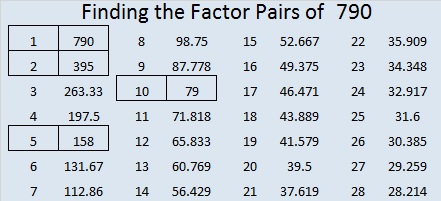# 790 and Level 3

• 790 is a composite number.
• Prime factorization: 790 = 2 x 5 x 79
• The exponents in the prime factorization are 1, 1, and 1. Adding one to each and multiplying we get (1 + 1)(1 + 1)(1 + 1) = 2 x 2 x 2 = 8. Therefore 790 has exactly 8 factors.
• Factors of 790: 1, 2, 5, 10, 79, 158, 395, 790
• Factor pairs: 790 = 1 x 790, 2 x 395, 5 x 158, or 10 x 79
• 790 has no square factors that allow its square root to be simplified. √790 ≈ 28.106939.Here is today’s puzzle:Print the puzzles or type the solution on this excel file: 10-factors-788-794

—————————

Here’s a little more about the number 790:

There are four ways to make 790 using three squares:

• 21² + 18² + 5² = 790
• 27² + 6² + 5² = 790
• 23² + 15² + 6² = 790
• 22² + 15² + 9² = 790

790 is the hypotenuse of Pythagorean triple 474-632-790 which is 3-4-5 times 158.

————————-This site uses Akismet to reduce spam. Learn how your comment data is processed.